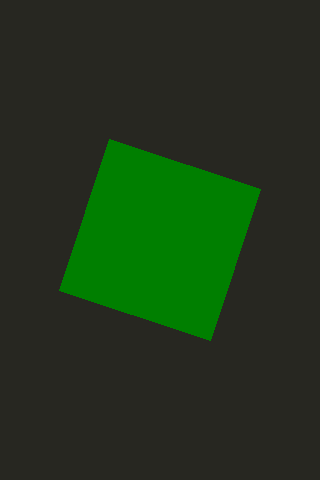# Use Case - Integrating JavaScript in QML

JavaScript code can be easily integrated into QML to provide UI logic, imperative control, or other benefits.

## Using JavaScript Expressions for Property Values

JavaScript expressions can be used in QML as bindings. For example:

```Item {
width: Math.random()
height: width < 100 ? 100 : (width + 50) /  2
}```

Note that function calls, like Math.random(), will not be reevaluated unless their arguments change. So binding to Math.random() will be one random number and not reevaluated, but if the width is changed in some other manner, the height binding will be reevaluated to take that into account.

## Adding JavaScript Functions in QML

JavaScript functions can be declared on QML items, like in the below example. This allows you to call the method using the item id.

```import QtQuick

Item {
id: container
width: 320
height: 480

function randomNumber() {
return Math.random() * 360;
}

function getNumber() {
return container.randomNumber();
}

TapHandler {
// This line uses the JS function from the item
onTapped: rectangle.rotation = container.getNumber();
}

Rectangle {
color: "#272822"
width: 320
height: 480
}

Rectangle {
id: rectangle
anchors.centerIn: parent
width: 160
height: 160
color: "green"
Behavior on rotation { RotationAnimation { direction: RotationAnimation.Clockwise } }
}

}```

## Using JavaScript Files

JavaScript files can be used for abstracting out logic from QML files. To do this, first place your functions inside a .js file like in the example shown.

```// myscript.js
function getRandom(previousValue) {
return Math.floor(previousValue + Math.random() * 90) % 360;
}```

Then import the file into any .qml file that needs to use the functions, like the example QML file below.

```import QtQuick
import "myscript.js" as Logic

Item {
width: 320
height: 480

Rectangle {
color: "#272822"
width: 320
height: 480
}

TapHandler {
// This line uses the JS function from the separate JS file
onTapped: rectangle.rotation = Logic.getRandom(rectangle.rotation);
}

Rectangle {
id: rectangle
anchors.centerIn: parent
width: 160
height: 160
color: "green"
Behavior on rotation { RotationAnimation { direction: RotationAnimation.Clockwise } }
}

}```For further details on the JavaScript engine used by QML, as well as the difference from browser JS, see the full documentation on JavaScript Expressions in QML Documents.